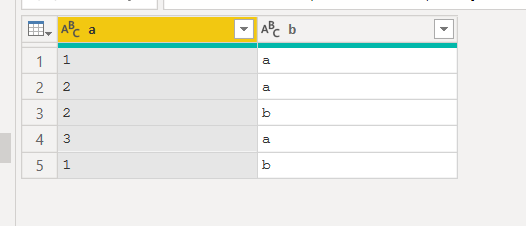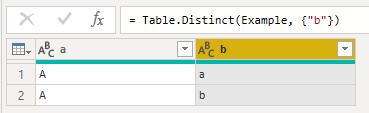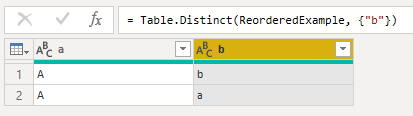cancel
Showing results for
Did you mean:## Which row will keep Table.Distinct(Table, {"Column"})

Hi colleagues,

Which row will keep Table.Distinct(Table, {"Column"}) for the rows with the same values in the "Column":

1. First row?
2. Last row?
3. Random row?

MS documentation has no explanation about it (https://docs.microsoft.com/en-us/powerquery-m/table-distinct)

19 REPLIES 19Super User

Hi @Belin ,
did you buffer the sort operation? (see here:  Bug warning for Table.Sort and removing duplicates... - Microsoft Power BI Community )

Imke Feldmann (The BIccountant)

If you liked my solution, please give it a thumbs up. And if I did answer your question, please mark this post as a solution. Thanks!Frequent Visitor

that's interesting, thank you.

At the end I used this

works like a charm

but I jsut noticed that in the notes she added a second better method, which I didn't have the time to try yetFrequent Visitor

I just found out the hard way.

the algorithm goies top to bottom, so I having a set like this

number updated_on

inc0001 01/01/22

inc0001 02/01/22

would remove the second occurrence from the top.

I wanted to keep the most updated record, so i sorted the data first, but still the algorithm remove the same  record, ignoring any sorting

At the end I'm 99% positive that eliminate from top to bottom every reoccurrence...hoping that is not using a something faster and more difficult to predict and control.

In the past the same function in excel worked this way, so I hope is still the same

CheersMicrosoft

https://docs.microsoft.com/en-us/power-query/commonissues#preserving-sortMicrosoft

I just wanted to let you know I've updated the docs page above based on this thread. Hopefully it's more relevant/helpful now.Regular Visitor

[SOLUTION]

After googling a lot, I fount this site explaining exactly what happened, and how to solve.

It’s simple, you just need add the buffer of the column you want to sort.

``= Table.Buffer(Table.Sort( #"Linhas Classificadas",{{"data_lancamento", Order.Descending}}))``Super User

You are forgetting that if you want to remove distinct letters that are different cases (A/a) then you need to add the optional parameter Comparer.OrdinalIgnoreCase

Table.Distinct(Table, {{“b”,Comparer.OrdinalIgnoreCase}})

This will treat A and a as duplicates. Otherwise, A/a are not the same in this function.
--Nate

I’m usually answering from my phone, which means the results are visualized only in my mind. You’ll need to use my answer to know that it works—but it will work!!Ok, this is pretty weird.

I ran the tests again, but instead of reordering the table using Table.Sort I just entered the data in a different order from the start.

Here are the results:

(For some reason I can't embed images even though I had no such problem yesterday. Sorry if this is harder to follow or the images eventually go down)

Source

Distinct

Reordered Source

Reordered Distinct

Code:

``````let
Source = Table.FromRows(Json.Document(Binary.Decompress(Binary.FromText("i45WclLSUUpUitWJVnJEYSUpxcYCAA==", BinaryEncoding.Base64), Compression.Deflate)), let _t = ((type nullable text) meta [Serialized.Text = true]) in type table [a = _t, b = _t]),
Example = Table.TransformColumnTypes(Source,{{"a", type text}, {"b", type text}}),
ExampleResult = Table.Distinct(Example, {"b"}),
ReorderedExample = Table.Sort(Example,{{"a", Order.Ascending}}),
ReorderedExampleResult = Table.Distinct(ReorderedExample, {"b"})
in
ReorderedExampleResult``````

We can easily see that order is indeed important, but that for some reason reordering the table using Table.Sort doesn't make a difference. I found that if we need to, we can use Table.Buffer to make Table.Distinct take into account the changes made by Table.Sort. Here's my third and final test:

Source

Distinct

Reordered Source

Reordered Distinct

Code:

``````let
Source = Table.FromRows(Json.Document(Binary.Decompress(Binary.FromText("i45WclTSUUpUitWJVnKCs0BiSUqxsQA=", BinaryEncoding.Base64), Compression.Deflate)), let _t = ((type nullable text) meta [Serialized.Text = true]) in type table [a = _t, b = _t]),
Example = Table.TransformColumnTypes(Source,{{"a", type text}, {"b", type text}}),
ExampleResult = Table.Distinct(Example, {"b"}),
ReorderedExample = Table.Buffer( Table.Sort(Example,{{"a", Order.Descending}}) ),
ReorderedExampleResult = Table.Distinct(ReorderedExample, {"b"})
in
ReorderedExampleResult``````Frequent Visitor

Thanks! This was a big help.Frequent Visitor

Thank you for doing the work of looking into this and identifying a solution to this frustrating issue.Anonymous
Not applicable

it seems as if the sort operation doesn't change the table from the point of view of the table.distinct function and uses the same result as before.(*)
The operations that "change" the table (manual reordering, table.buffer, adding an index column, replacing any value in the table - even if the new value is the same as the old one -, changing the type of a column) do " start " the procedure of the table.distinct function all over again

(*) if between the call to the table.distinct function and the definition of the table to which it is applied, only table.sort functions act, the table distinct function is applied to the original table "saving" the sorting operation which is generally heavy.
Maybe ‌ 😁Anonymous
Not applicable

table.group also appears to have similar behavior to table.distinct.
After all, a possible algorithm to eliminate duplicates in pseudocode could be like this:

Table.Group (tab, "col", each Table.First (_) [all columns but "col"])

``````let
Source = Table.FromRows(Json.Document(Binary.Decompress(Binary.FromText("i45WMlTSUUpUitWJVjJCYSWBWcZwMUOIWCwA", BinaryEncoding.Base64), Compression.Deflate)), let _t = ((type nullable text) meta [Serialized.Text = true]) in type table [a = _t, b = _t]),
Example = Table.TransformColumnTypes(Source,{{"a", type text}, {"b", type text}}),
#"Raggruppate righe" = Table.Group(Example, {"b"}, {{"all", each Table.First(_)[a]}}),
Example1 = Table.TransformColumnTypes(Source,{{"a", type text}, {"b", type text}}),
#"Ordinate righe" = Table.Sort(Example1,{{"a", Order.Descending}}),
#"Raggruppate righe1" = Table.Group(#"Ordinate righe", {"b"}, {{"all", each List.First(_[a]) }})
in
#"Raggruppate righe1"``````

in the following form the "problem" doesn't arise:

``````let
Source = Table.FromRows(Json.Document(Binary.Decompress(Binary.FromText("i45WMlTSUUpUitWJVjJCYSWBWcZwMUOIWCwA", BinaryEncoding.Base64), Compression.Deflate)), let _t = ((type nullable text) meta [Serialized.Text = true]) in type table [a = _t, b = _t]),
Example = Table.TransformColumnTypes(Source,{{"a", type text}, {"b", type text}}),
#"Raggruppate righe" = Table.Group(Example, {"b"}, {{"all", each _}}),
Example1 = Table.TransformColumnTypes(Source,{{"a", type text}, {"b", type text}}),
#"Ordinate righe" = Table.Sort(Example1,{{"a", Order.Descending}}),
#"Raggruppate righe1" = Table.Group(#"Ordinate righe", {"b"}, {{"all", each _ }})
in
#"Raggruppate righe1"``````

and not even like this

``````let
Source = Table.FromRows(Json.Document(Binary.Decompress(Binary.FromText("i45WMlTSUUpUitWJVjJCYSWBWcZwMUOIWCwA", BinaryEncoding.Base64), Compression.Deflate)), let _t = ((type nullable text) meta [Serialized.Text = true]) in type table [a = _t, b = _t]),
Example = Table.TransformColumnTypes(Source,{{"a", type text}, {"b", type text}}),
#"Raggruppate righe" = Table.Group(Example, {"b"}, {{"all", each _[a]{0}}}),
Example1 = Table.TransformColumnTypes(Source,{{"a", type text}, {"b", type text}}),
#"Ordinate righe" = Table.Sort(Example1,{{"a", Order.Descending}}),
#"Raggruppate righe1" = Table.Group(#"Ordinate righe", {"b"}, {{"all", each _[a]{0} }})
in
#"Raggruppate righe1"``````Anonymous
Not applicable

Suppose you have this table:if you sort by column a, you get this:but even this different situation would have been valid (third and fourth row are reversed):This, I believe, depends on the internal algorithm used to do the sorting.

If, as plausible as it may be, the table.distinct algorithm first does a sort of rows to "group" the rows that have duplicate values in the control columns, the ordering of the remaining columns, as we have seen, is not determined.

So if the algorithm also takes the first row of the group, it is not necessarily the "first" row you encounter  the source table.Helper IV

Hello,

I think this is similar to my issue:

https://community.powerbi.com/t5/Desktop/Power-Query-Row-disappear-after-expand-column/m-p/929805#M4...

It is intended behaviour - Power query steps are not always performed one-by-one. You can force one-by-one by using Table.BufferMicrosoft

I believe it keeps the first one.  You can do quick try to confirm.  You can add a sort step just before that step to keep the one you want.

If this works for you, please mark it as the solution.  Kudos are appreciated too.  Please let me know if not.

Regards,

Pat

Did I answer your question? Mark my post as a solution! Kudos are also appreciated!Hi @mahoneypatI, I also "believe" in "First row", but it is good to have official confirmation from MS. Isn't it? 🙂Super User

Just be aware that if you have to sort your table to define the desired logic, you have to use a Table.Buffer with it, as the sort order might not stick without it (mostly relevant for large tables): Bug warning for Table.Sort and removing duplicates... - Microsoft Power BI Community

Imke Feldmann (The BIccountant)

If you liked my solution, please give it a thumbs up. And if I did answer your question, please mark this post as a solution. Thanks!Community Support

Sorry for that, but we did not find any logic definition of this function in official document. But you can confirm it by the Example 2 of the function document, it will keep the first row.Best regards,

Community Support Team _ Dong Li
If this post helps, then please consider Accept it as the solution to help the other members find it more quickly.Hey @v-lid-msft , I did a quick test and that does not seem to be the case.

First, I entered the data from that exampleand used 'Remove duplicates' on bThis is what we expected from the documentation and from the assumption that it keeps the first row.

Now to the real test. I altered the order of the first table so that it looks like this:Under the assumption that it keeps the first row, we expect to get the following table:

 a b B a A bHere's the code for the tests in case anyone wants to check

``````let
Source = Table.FromRows(Json.Document(Binary.Decompress(Binary.FromText("i45WclTSUUpUitWJVnKCs0BiSUqxsQA=", BinaryEncoding.Base64), Compression.Deflate)), let _t = ((type nullable text) meta [Serialized.Text = true]) in type table [a = _t, b = _t]),
Example = Table.TransformColumnTypes(Source,{{"a", type text}, {"b", type text}}),
ExampleResult = Table.Distinct(Example, {"b"}),
ReorderedExample = Table.Sort(Example,{{"a", Order.Descending}}),
ReorderedExampleResult = Table.Distinct(ReorderedExample, {"b"})
in
ReorderedExampleResult``````